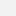# Humboldt-Universität zu Berlin - Statistik

Computergestützte Statistik W (VL)

Kategorie
Bachelor
Lehrende(r)

# Course Outline

Today almost all statistical data analyses are carried out with the aid of the computer, in conjunction with a software package. However, computer-based data analysis requires substantial knowledge in statistics to select appropriate statistical methods and models for the special professional problem of interest, taking account of their assumptions, and to draw correct conclusions from the computer output.Therefore, computational statistics is devoted to basic statistical theory and concepts in conjunction with computing methods. In the course "Computer assisted Statistics" the software package SPSS for Windows is used. Special knowledge in a programming language is not required for handling SPSS.

The first part of the course is devoted to a short overlook of data handling, data selection and data transformation in the SPSS system. The evaluation of data usually starts with univariate studies and terminates with multivariate ones. In each step of the evaluation different statistical concepts will be used: exploration, description and inference. The course follows this outline.

##### The course focuses on three statistical topics:
• Discovery and identification of outliers

Observed data sets contain very often so-called atypical values (outliers) which may seriously effect the results of applied statistical methods. Exploratory techniques, especially graphical tools (stem-and-leaf-plot, boxplot, scatterplot), are used to discover and to identify potential outliers. Tests for outliers are considered. On the accommodation of outliers in the data set, some estimation procedures of the population mean are introduced which are robust in the sense of providing protection against the effect of outliers.

• Hypothesis tests about sampling distributions

Statistical inference mostly depends on some assumptions on the population distribution. The validity of these assumptions has to be checked using the sample observations. Graphical tools (histogram, probability plots) and descriptive measures are applied to get a first impression of the sample distribution. Goodness-of-fit tests are appropriate for testing the fit of a theoretical distribution to observed data (Kolmogorov-Smirnov-Test, Chi-Square-Test of Goodness-of Fit, Binomial-Test). For continuous variables one of the most important assumption to be tested is whether the normal distribution is a good fit of the data.

• Hypothesis tests about differences between population parameters

Often information on more than one variable for each element are available, so that the variable of interest can be grouped according to the outcomes of the other variable, e.g. household net income grouped by size of household, income grouped by sex. This part of the course extends the discussion to the comparison of parameters of several populations. We will focus on the comparison of the population means by using exploratory tools and hypothesis tests.

# Literature

• Barnett, V., Lewis, T. (1994) Outliers in statistical data, 3rd. Edition, Wiley, New York
• Berry, D.A., Lindgren, B.W. (1990), Statistics: Theory and Methods, Brooks/Cole Publishing Company, Pacific Grove
• Bortz, J. (1993), Statistik, Springer, Berlin et al.
• Bosch, K. (1992), Statistik-Taschenbuch, Oldenbourg, München, Wien
• Bühl, A., Zöfel, P. (1994), SPSS unter Windows Version 6, Addison-Wesley, Bonn et al.
• Böning, H., Trenkler, G. (1978), Nichtparametrische statistische Methoden, Walter de Gruyter, Berlin, New York
• Böning, H. (1991), Robuste und adaptive Tests, Walter de Gruyter, Berlin, New York
• Hartung, J., Elpelt, B., Klösener, K.-H. (1993), Statistik, Oldenbourg Verlag, München
• Heiler, S., Michels, P. (1994), Deskriptive und explorative Datenanalyse, Oldenbourg, München, Wien
• Jobson, J.D. (1991), Applied Multivariate Data Analysis, Vol. I: Regression and Experimental Design, Springer, Berlin et al.
• Köhler, W.-M. (1994), SPSS für Windows, Vieweg, Wiesbaden
• Mann, P. S. (1992), Introductory Statistics, John Wiley, New York at al.
• Rasmussen, S. (1992), An Introduction to Statistics with Data Analysis, Brooks/Cole Publishing Company, Pacific Grove
• Rönz: Skript zur Vorlesung "Computergestützte Statistik I", 2001
• Rönz, B., Strohe, H. G. (Hrsg., 1994), Lexikon Statistik, Gabler-Verlag, Wiesbaden
• Schlittgen, R. (1990), Einführung in die Statistik, Oldenbourg, München, Wien